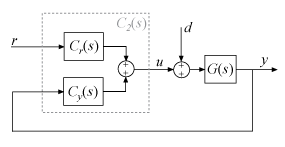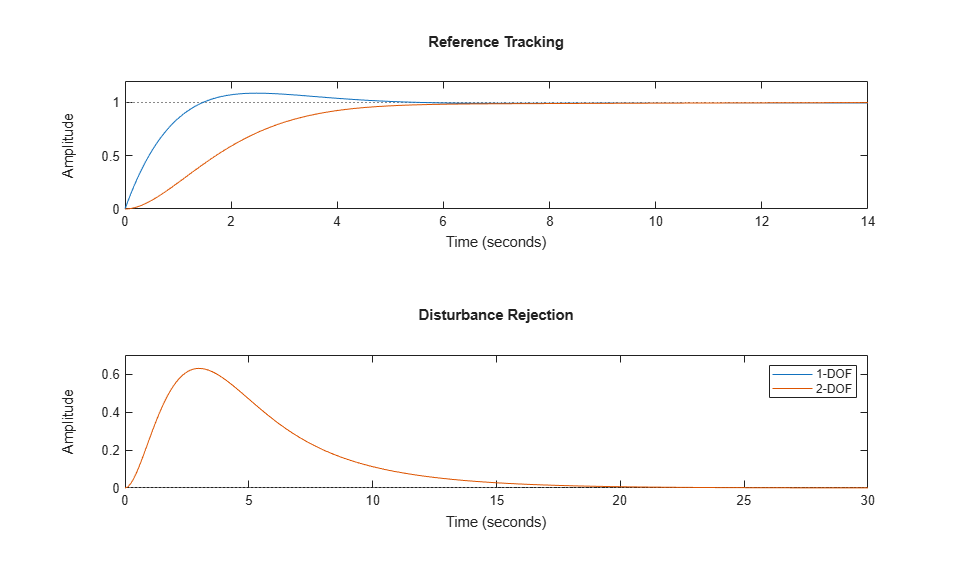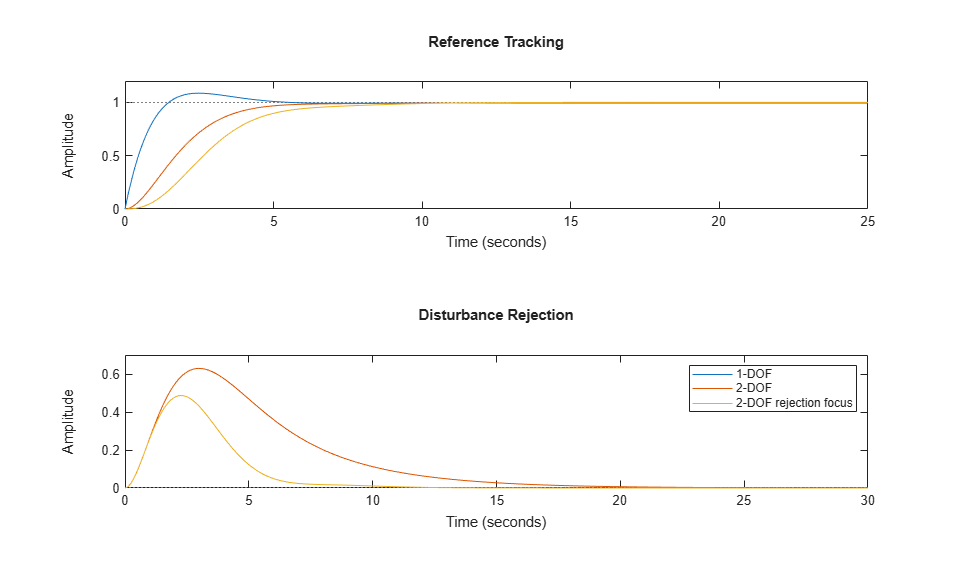Documentation

## Tune 2-DOF PID Controller (Command Line)

This example shows how to design a two-degree-of-freedom (2-DOF) PID controller at the command line. The example also compares the 2-DOF controller performance to the performance achieved with a 1-DOF PID controller.

2-DOF PID controllers include setpoint weighting on the proportional and derivative terms. Compared to a 1-DOF PID controller, a 2-DOF PID controller can achieve better disturbance rejection without significant increase of overshoot in setpoint tracking. A typical control architecture using a 2-DOF PID controller is shown in the following diagram.For this example, design a 2-DOF controller for the plant given by:

``

```wc = 1.5; G = tf(1,[1 0.5 0.1]); C2 = pidtune(G,'PID2',wc) ```
```C2 = 1 u = Kp (b*r-y) + Ki --- (r-y) + Kd*s (c*r-y) s with Kp = 1.26, Ki = 0.255, Kd = 1.38, b = 0.665, c = 0 Continuous-time 2-DOF PID controller in parallel form. ```

Using the type `'PID2'` causes `pidtune` to generate a 2-DOF controller, represented as a `pid2` object. The display confirms this result. `pidtune` tunes all controller coefficients, including the setpoint weights `b` and `c`, to balance performance and robustness.

To compute the closed-loop response, note that a 2-DOF PID controller is a 2-input, 1-output dynamic system. You can resolve the controller into two channels, one for the reference signal and one for the feedback signal, as shown in the diagram. (See Continuous-Time 2-DOF PID Controller Representations for more information.)Decompose the controller into the components `Cr` and `Cy`, and use them to compute the closed-loop response from r to y.

```C2tf = tf(C2); Cr = C2tf(1); Cy = C2tf(2); T2 = Cr*feedback(G,Cy,+1); ```

To examine the disturbance-rejection performance, compute the transfer function from d to y.

```S2 = feedback(G,Cy,+1); ```

For comparison, design a 1-DOF PID controller with the same bandwidth and compute the corresponding transfer functions. Then compare the step responses.

```C1 = pidtune(G,'PID',wc); T1 = feedback(G*C1,1); S1 = feedback(G,C1); subplot(2,1,1) stepplot(T1,T2) title('Reference Tracking') subplot(2,1,2) stepplot(S1,S2) title('Disturbance Rejection') legend('1-DOF','2-DOF') ```The plots show that adding the second degree of freedom eliminates the overshoot in the reference-tracking response without any cost to disturbance rejection. You can improve disturbance rejection too using the `DesignFocus` option. This option causes `pidtune` to favor disturbance rejection over setpoint tracking.

```opt = pidtuneOptions('DesignFocus','disturbance-rejection'); C2dr = pidtune(G,'PID2',wc,opt) ```
```C2dr = 1 u = Kp (b*r-y) + Ki --- (r-y) + Kd*s (c*r-y) s with Kp = 1.72, Ki = 0.593, Kd = 1.25, b = 0, c = 0 Continuous-time 2-DOF PID controller in parallel form. ```

With the default balanced design focus, `pidtune` selects a `b` value between 0 and 1. For this plant, when you change design focus to favor disturbance rejection, `pidtune` sets `b` = 0 and `c` = 0. Thus, `pidtune` automatically generates an I-PD controller to optimize for disturbance rejection. (Explicitly specifying an I-PD controller without setting the design focus yields a similar controller.)

Compare the closed-loop responses using all three controllers.

```C2dr_tf = tf(C2dr); Cdr_r = C2dr_tf(1); Cdr_y = C2dr_tf(2); T2dr = Cdr_r*feedback(G,Cdr_y,+1); S2dr = feedback(G,Cdr_y,+1); subplot(2,1,1) stepplot(T1,T2,T2dr) title('Reference Tracking') subplot(2,1,2) stepplot(S1,S2,S2dr); title('Disturbance Rejection') legend('1-DOF','2-DOF','2-DOF rejection focus') ```The plots show that the disturbance rejection is further improved compared to the balanced 2-DOF controller. This improvement comes with some sacrifice of reference-tracking performance, which is slightly slower. However, the reference-tracking response still has no overshoot.

Thus, using 2-DOF control can improve disturbance rejection without sacrificing as much reference tracking performance as 1-DOF control. These effects on system performance depend strongly on the properties of your plant. For some plants and some control bandwidths, using 2-DOF control or changing the design focus has less or no impact on the tuned result.